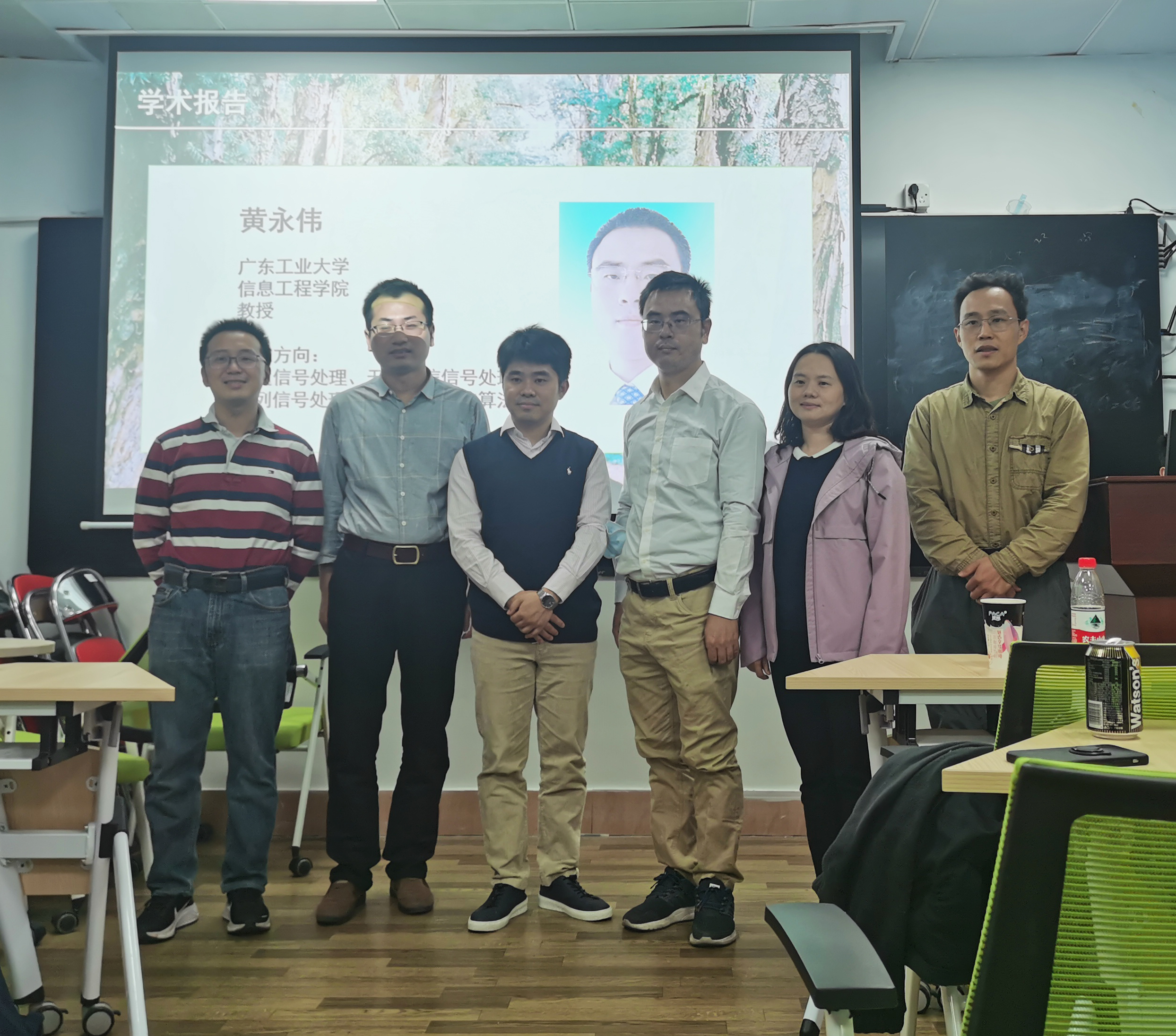# 黄永伟教授受邀进行讲座

黄博士现为IEEE高级会员。现任“Signal Processing”和“ IEEE Transactions on Signal and Information Processing over Networks”杂志编委。现任IEEE信号处理协会“传感器阵列与多通道信号处理”专业技术委员会委员。曾任IEEE杂志“IEEE Signal Processing Letters”编委（2016-2020）。曾于2009年访问意大利那不勒斯“腓特烈二世”大学，并为该校工学院研究生开设“凸优化理论与应用”暑期课程。黄博士的研究兴趣包括：最优化算法与分析，阵列、雷达、无线通信信号处理等。

The worst-case robust adaptive beamforming problem for general-rank signal model is considered. This is a nonconvex problem, and an approximate version of it (obtained by introducing a matrix decomposition on the presumed covariance matrix of the desired signal) has been well studied in the literature. Different from the existing literature, herein however the original beamforming problem is tackled. Resorting to the strong duality of linear conic programming, the robust adaptive beamforming problem for general-rank signal model is reformulated into an equivalent quadratic matrix inequality (QMI) problem. By employing a linear matrix inequality (LMI) relaxation technique, the QMI problem is turned into a convex semidefinite programming problem. Using the fact that there is often a positive gap between the QMI problem and its LMI relaxation, an approximation algorithm is proposed to solve the robust adaptive beamforming in the QMI form. Besides, several sufficient optimality conditions for the nonconvex QMI problem are developed. To validate our results, simulation examples are presented, which demonstrate the improved performance of the new robust beamformer in terms of the output signal-to-interference-plus-noise ratio.